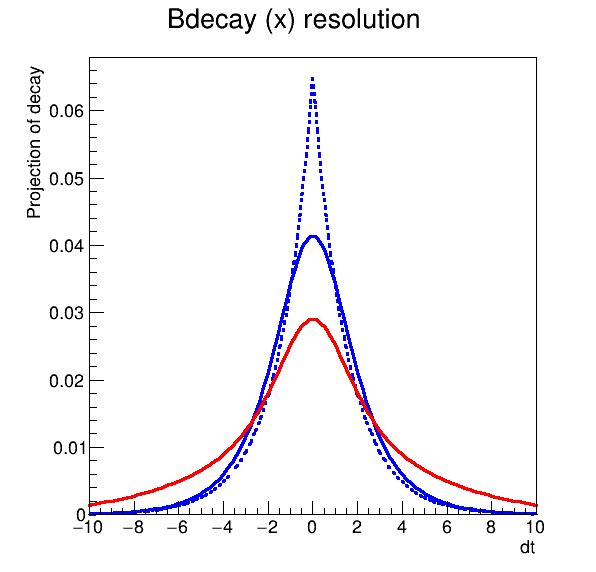# Rf 2 0 9_Anaconv¶

Addition and convolution: decay function pdfs with optional B physics effects (mixing and CP violation) that can be analytically convolved with e.g. Gaussian resolution functions

pdf1 = decay(t,tau) (x) delta(t)
pdf2 = decay(t,tau) (x) gauss(t,m,s)
pdf3 = decay(t,tau) (x) (f*gauss1(t,m1,s1) + (1-f)*gauss2(t,m1,s1))

Author: Clemens Lange, Wouter Verkerke (C++ version)
This notebook tutorial was automatically generated with ROOTBOOK-izer from the macro found in the ROOT repository on Saturday, November 28, 2020 at 10:42 AM.

In :
import ROOT

Welcome to JupyROOT 6.23/01


## B-physics pdf with truth resolution¶

Variables of decay pdf

In :
dt = ROOT.RooRealVar("dt", "dt", -10, 10)
tau = ROOT.RooRealVar("tau", "tau", 1.548)

RooFit v3.60 -- Developed by Wouter Verkerke and David Kirkby
Copyright (C) 2000-2013 NIKHEF, University of California & Stanford University



Build a truth resolution model (delta function)

In :
tm = ROOT.RooTruthModel("tm", "truth model", dt)


Construct decay(t) (x) delta(t)

In :
decay_tm = ROOT.RooDecay("decay_tm", "decay", dt,
tau, tm, ROOT.RooDecay.DoubleSided)


Plot pdf (dashed)

In :
frame = dt.frame(ROOT.RooFit.Title("Bdecay (x) resolution"))
decay_tm.plotOn(frame, ROOT.RooFit.LineStyle(ROOT.kDashed))

Out:
<cppyy.gbl.RooPlot object at 0x75c6e20>

## B-physics pdf with Gaussian resolution¶

Build a gaussian resolution model

In :
bias1 = ROOT.RooRealVar("bias1", "bias1", 0)
sigma1 = ROOT.RooRealVar("sigma1", "sigma1", 1)
gm1 = ROOT.RooGaussModel("gm1", "gauss model 1", dt, bias1, sigma1)


Construct decay(t) (x) gauss1(t)

In :
decay_gm1 = ROOT.RooDecay("decay_gm1", "decay",
dt, tau, gm1, ROOT.RooDecay.DoubleSided)


Plot pdf

In :
decay_gm1.plotOn(frame)

Out:
<cppyy.gbl.RooPlot object at 0x75c6e20>

## B-physics pdf with double Gaussian resolution¶

Build another gaussian resolution model

In :
bias2 = ROOT.RooRealVar("bias2", "bias2", 0)
sigma2 = ROOT.RooRealVar("sigma2", "sigma2", 5)
gm2 = ROOT.RooGaussModel("gm2", "gauss model 2", dt, bias2, sigma2)


Build a composite resolution model fgm1+(1-f)gm2

In :
gm1frac = ROOT.RooRealVar("gm1frac", "fraction of gm1", 0.5)
"gmsum",
"sum of gm1 and gm2",
ROOT.RooArgList(
gm1,
gm2),
ROOT.RooArgList(gm1frac))


Construct decay(t) (x) (fgm1 + (1-f)gm2)

In :
decay_gmsum = ROOT.RooDecay(
"decay_gmsum", "decay", dt, tau, gmsum, ROOT.RooDecay.DoubleSided)


Plot pdf (red)

In :
decay_gmsum.plotOn(frame, ROOT.RooFit.LineColor(ROOT.kRed))

Out:
<cppyy.gbl.RooPlot object at 0x75c6e20>

Draw all frames on canvas

In :
c = ROOT.TCanvas("rf209_anaconv", "rf209_anaconv", 600, 600)
frame.GetYaxis().SetTitleOffset(1.6)
frame.Draw()

c.SaveAs("rf209_anaconv.png")

Info in <TCanvas::Print>: png file rf209_anaconv.png has been created


Draw all canvases

In :
from ROOT import gROOT
gROOT.GetListOfCanvases().Draw()